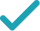Type a math problemSolve for xSteps Using Factoring
Steps Using Factoring By Grouping
Steps for Completing the Square
Steps Using Factoring
Subtract from both sides.
To solve the equation, factor using formula . To find and , set up a system to be solved.
Since is negative, and have the opposite signs. Since is negative, the negative number has greater absolute value than the positive. List all such integer pairs that give product .
Calculate the sum for each pair.
The solution is the pair that gives sum .
Rewrite factored expression using the obtained values.
To find equation solutions, solve and .
GraphGraph Both Sides in 2D
Graph in 2D
x^{2}-3x-28=0
Subtract 28 from both sides.
a+b=-3 ab=-28
To solve the equation, factor x^{2}-3x-28 using formula x^{2}+\left(a+b\right)x+ab=\left(x+a\right)\left(x+b\right). To find a and b, set up a system to be solved.
1,-28 2,-14 4,-7
Since ab is negative, a and b have the opposite signs. Since a+b is negative, the negative number has greater absolute value than the positive. List all such integer pairs that give product -28.
1-28=-27 2-14=-12 4-7=-3
Calculate the sum for each pair.
a=-7 b=4
The solution is the pair that gives sum -3.
\left(x-7\right)\left(x+4\right)
Rewrite factored expression \left(x+a\right)\left(x+b\right) using the obtained values.
x=7 x=-4
To find equation solutions, solve x-7=0 and x+4=0.
x^{2}-3x-28=0
Subtract 28 from both sides.
a+b=-3 ab=1\left(-28\right)=-28
To solve the equation, factor the left hand side by grouping. First, left hand side needs to be rewritten as x^{2}+ax+bx-28. To find a and b, set up a system to be solved.
1,-28 2,-14 4,-7
Since ab is negative, a and b have the opposite signs. Since a+b is negative, the negative number has greater absolute value than the positive. List all such integer pairs that give product -28.
1-28=-27 2-14=-12 4-7=-3
Calculate the sum for each pair.
a=-7 b=4
The solution is the pair that gives sum -3.
\left(x^{2}-7x\right)+\left(4x-28\right)
Rewrite x^{2}-3x-28 as \left(x^{2}-7x\right)+\left(4x-28\right).
x\left(x-7\right)+4\left(x-7\right)
Factor out x in the first and 4 in the second group.
\left(x-7\right)\left(x+4\right)
Factor out common term x-7 by using distributive property.
x=7 x=-4
To find equation solutions, solve x-7=0 and x+4=0.
x^{2}-3x=28
All equations of the form ax^{2}+bx+c=0 can be solved using the quadratic formula: \frac{-b±\sqrt{b^{2}-4ac}}{2a}. The quadratic formula gives two solutions, one when ± is addition and one when it is subtraction.
x^{2}-3x-28=28-28
Subtract 28 from both sides of the equation.
x^{2}-3x-28=0
Subtracting 28 from itself leaves 0.
x=\frac{-\left(-3\right)±\sqrt{\left(-3\right)^{2}-4\left(-28\right)}}{2}
This equation is in standard form: ax^{2}+bx+c=0. Substitute 1 for a, -3 for b, and -28 for c in the quadratic formula, \frac{-b±\sqrt{b^{2}-4ac}}{2a}.
x=\frac{-\left(-3\right)±\sqrt{9-4\left(-28\right)}}{2}
Square -3.
x=\frac{-\left(-3\right)±\sqrt{9+112}}{2}
Multiply -4 times -28.
x=\frac{-\left(-3\right)±\sqrt{121}}{2}
x=\frac{-\left(-3\right)±11}{2}
Take the square root of 121.
x=\frac{3±11}{2}
The opposite of -3 is 3.
x=\frac{14}{2}
Now solve the equation x=\frac{3±11}{2} when ± is plus. Add 3 to 11.
x=7
Divide 14 by 2.
x=\frac{-8}{2}
Now solve the equation x=\frac{3±11}{2} when ± is minus. Subtract 11 from 3.
x=-4
Divide -8 by 2.
x=7 x=-4
The equation is now solved.
x^{2}-3x=28
Quadratic equations such as this one can be solved by completing the square. In order to complete the square, the equation must first be in the form x^{2}+bx=c.
x^{2}-3x+\left(-\frac{3}{2}\right)^{2}=28+\left(-\frac{3}{2}\right)^{2}
Divide -3, the coefficient of the x term, by 2 to get -\frac{3}{2}=-1.5. Then add the square of -\frac{3}{2}=-1.5 to both sides of the equation. This step makes the left hand side of the equation a perfect square.
x^{2}-3x+\frac{9}{4}=28+\frac{9}{4}
Square -\frac{3}{2}=-1.5 by squaring both the numerator and the denominator of the fraction.
x^{2}-3x+\frac{9}{4}=\frac{121}{4}